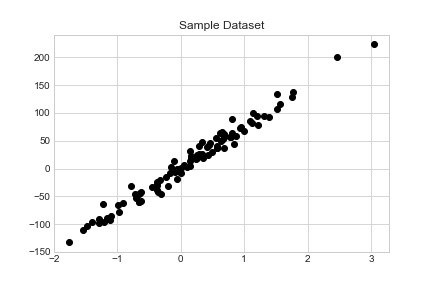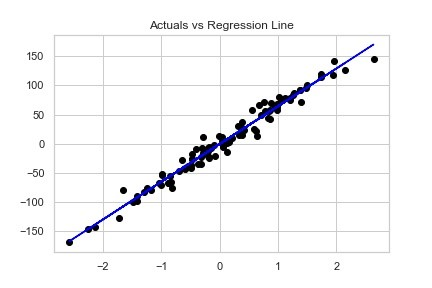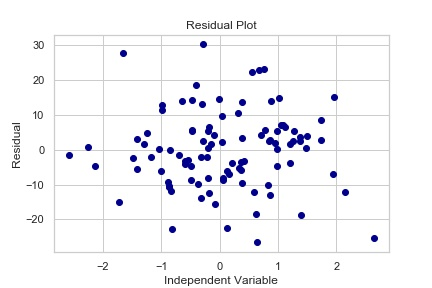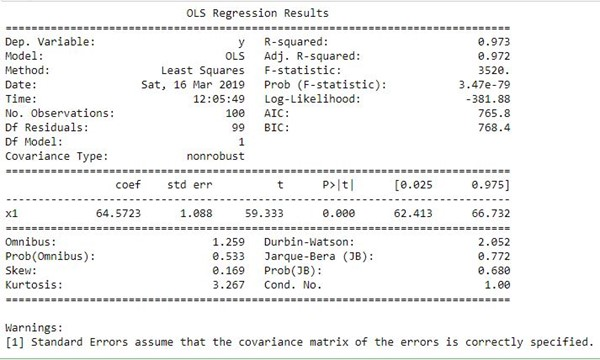Python Linear Regression Analysis

When embarking on a data science learning path, regression analysis is one of the first predictive algorithms that you learn. It is widely used throughout statistics and business. It is definitely a tool you must have in your data science arsenal. In this article we will show you how to conduct a linear regression analysis using python.

What is Regression Analysis?

Regression analysis is a widely used and powerful statistical technique to quantify the relationship between 2 or more variables.

Most commonly, it is used to explain the relationship between an independent and dependent variables. The dependent variable is what you are trying to predict while your inputs become your independent variables.

For example, if we have a data set of revenue and price and we are trying to quantify what happens to revenue when we change the price. Price becomes your independent variable, revenue (what you are trying to predict) is your dependent variable.

Assumptions of Linear Regression

In order to correctly apply linear regression, you must meet these 5 key assumptions:

• We are investigating a linear relationship
• All variables follow a normal distribution
• There is very little or no multicollinearity
• There is little or no autocorrelation
• Data is homoscedastic

To understand more about these assumptions and how to test them using Python, read this article: Assumptions of Linear Regression with Python

Linear Regression Formula

When performing a regression analysis, the goal is to generate an equation that explains the relationship of your independent and dependent variables.

In a linear regression, the equation follows the below.

In which m is the slope of the line, b is the point at which the regression line intercepts the y axis. X is the independent variable.

How do we get the coefficients and intercepts you ask? This is where we will use python’s statistical packages to do the hard work for us.

Sklearn Linear Regression

Let’s see how we can come up with the above formula using the popular python package for machine learning, Sklearn.

First, generate some data that we can run linear regression on.

# generate regression dataset
from sklearn.datasets.samples_generator import make_regression
X, y = make_regression(n_samples=100, n_features=1, noise=10)

Second, create a scatter plot to visualize the relationship.

%matplotlib inline
import matplotlib.pyplot as plt
plt.style.use('seaborn-whitegrid')

plt.plot(X, y, 'o', color='black');
plt.title("Sample Dataset"

Below we can clearly see there is a relationship between our independent and dependent variable.Now, use Sklearn to run regression analysis.

from sklearn.linear_model import LinearRegression
#run regression
reg = LinearRegression().fit(X, y)

Next, plot our fitted line against our dataset to visually see how well it fits.

#Generated Predictions
y_predicted = reg.predict(X)

#Plot Our Actual and Predicted Values
plt.plot(X, y, 'o', color='black');
plt.plot(X,y_predicted,color='blue')

plt.title("Actuals vs Regression Line")

It’s easy to see our regression fits our input data quite well.To get the coefficients and intercept is a matter of running the following code.

#get coefficients and y intercept
print("m: {0}".format(reg.coef_))
print("b: {0}".format(reg.intercept_))

#m: [64.61969623]
#b: -0.5324534814869875

R-Squared

A metric you can use to quantify how much dependent variable variation your linear model explains is called R-Squared (R2). In other words, it evaluates how closely y values scatter around your regression line, the closer they are to your regression line the better.

The range of R-Squared goes from 0% to 100%. The higher the R-Squared the better.

#Returns the coefficient of determination R^2 of the prediction.
reg.score(X, y)
#0.9725287282456724

In our case, our regression line is able to explain 97.25% of the variation, pretty good!

Be careful though, you can’t just use R-Squared to determine how good your model is. For example, your coefficients could be biased and you wouldn’t know by looking at R-Squared. And, if you have multiple independent variables it doesn’t tell you anything about them.

Residual Plots

Residuals are the difference between the dependent variable (y) and the predicted variable (y_predicted).

Residual plot is a scatter plot of the independent variables and the residual. Let’s calculate the residuals and plot them.

residuals = y-y_predicted
plt.plot(X,residuals, 'o', color='darkblue')
plt.title("Residual Plot")
plt.xlabel("Independent Variable")
plt.ylabel("Residual")

Visual inspection of these residual plots will let you know if you have bias in your independent variables and thus are breaking either the autocorrelation or homoscedastic assumptions of regression analysis.When analysing residual plot, you should see a random pattern of points. If you notice a trend in these plots, you could have an issue with your coefficients. In our plot above, there is no trend of the residuals.

Interpreting Regression Coefficients

This is an important step when performing a regression analysis. At the end of the day, the coefficients and intercepts are the values you are looking for in order to quantify the relationship. How do you know if the independent variable is truly predictive or not?

To interpret the regression coefficients you must perform a hypothesis test of the coefficients. In a regression analysis, it goes as follows:

• Null Hypothesis (H0): The coefficients are zero
• Alternate Hypothesis (H1): The coefficients are NOT zero

In other words, if the coefficients are truly zero, it means that independent variable has no predictive power and should be tossed away. This hypothesis test is performed on all coefficients.

To do this, let’s turn the the statsmodels package and run a linear regression analysis using the ordinary least squares model. Once complete, print the summary.

import statsmodels.api as sm

est = sm.OLS(y, X)
est2 = est.fit()
print(est2.summary())Upon closer inspection you will see the R-Squared we previously calculated with Sklearn of 97.3%.

To test the coefficients null hypothesis we will be using the t statistic. Look at the P>| t | column. These are the p-values for the t test. In short, if they are less than a desired significance (commonly .05), you reject the null hypothesis. Otherwise, you fail to reject the null and therefore should toss out that independent variable.

Above, assuming a significance value of 0.05, our P-Value of 0.000 is much lower than a significance. Therefore, we reject the null hypothesis that the coefficient is equal to 0 and conclude that x1 is an important independent variable to utilize.

If you had more independent variables they would be listed here and you would perform a similar test.

Conclusion

Regression analysis is an important statistical technique widely used throughout statistics and business. It is a must known tool in our data science toolkit. Now, you are armed with the knowledge of how to utilize python to perform linear regression analysis. Fitting the regression line and being able to interpret the results of how good of a model you have.